# Gate Mock Test- 2: CS/IT

## 65 Questions MCQ Test GATE Computer Science Engineering(CSE) 2023 Mock Test Series | Gate Mock Test- 2: CS/IT

Description
Attempt Gate Mock Test- 2: CS/IT | 65 questions in 180 minutes | Mock test for GATE preparation | Free important questions MCQ to study GATE Computer Science Engineering(CSE) 2023 Mock Test Series for GATE Exam | Download free PDF with solutions
QUESTION: 1

### A vendor sells his articles at a certain profit percentage. If he sells his articles at ¼th of his actual selling price then he incurs a loss of 60%. What is his actual profit percentage?

Solution:

Let the cost price = 100 Rs.

From the options:

If profit % = 60%

Then SP = 160 Rs.

New SP = 160/4 = 40 Rs.

Then,

Percentage loss= (100-40)/100 = 60%

Hence Verified

ALTERNATE:-

Let the selling price is 100

New selling price 100/4 = 25

He suffers a loss of 60%.

CP*0.4 = 25

CP = 25/0.4 = 62.5

Actual Profit = 100 - 62.5 = 37.5

profit% = 37.5/62.5 *100 = 60%

QUESTION: 2

### Find wrong number in series: 23, 29, 31, 33, 41, 43, 47

Solution:

the given series is prime numbers from 23

QUESTION: 3

### Criteria for selecting candidate for internship programmeThe candidate:(1) can preferably start the internship between 18th Oct'17 and 17th Nov'17(2) are preferably available for duration of 6 months(3) have computer skills and interest in designing(4) have already graduated or are currently in any year of study(5) knows to deal with customersNick is a high school student and wants to do an internship as his summer project. He is a very vibrant boy and goes well with people. Is he the right candidate for the internship?

Solution: He does is looking for summer internships and November is not summer time. Hence, option B is the correct option.
QUESTION: 4

Direction: In the given question, one statement with a blank along with four words is given. Two of the given words can fit into the given blank. Five options with various combinations of these words are given. Choose the combination of the words that best fits into the blank.

Comedian Vasu Primlani takes a hilarious ______ at the new trend of renaming Indian cities.

a) Jabs

b) Jokes

c) Satires

d) Gags

Solution: The meaning of the words as follows:

a. Jab as a noun refers to a quick, sharp blow, especially with the fist.

b. A joke is a thing that someone says to cause amusement or laughter, especially a story with a funny punchline.

c. Satire is the use of humour, irony, exaggeration, or ridicule to expose and criticize people's stupidity or vices, particularly in the context of contemporary politics and other topical issues.

d. A gag is a joke or an amusing story, especially one forming part of a comedian's act, or in a film.

‘Hilarious satires’ and ‘hilarious jabs’ seem inappropriate and ambiguous. Among all the options, ‘jokes’ and ‘gags’ fit perfectly in the blank. Therefore, option D is the apt answer.

QUESTION: 5

Which of the following is MOST OPPOSITE in meaning to Locus?

Solution: Locus (noun) -a particular position or place where something occurs or is situated.
QUESTION: 6

The ratio between the speed of a bus and train is 15 : 27, respectively. Also, a car covered a distance of 720 km in 9 h. The speed of the bus is three- fourth of the speed of the car. How much distance will the train cover at 7 h?

Solution:

speed of car = distance covered / time taken

720 / 9 = 80 km/h

Now, speed of bus = 3 / 4 x 80 = 60 km/h

Speed of train = 27 / 15 x 60 = 108 km/h

Distance covered by train in 7 h = 108 x 7 = 756 km

QUESTION: 7

The Union Sports Ministry has approved five lakh rupees from the National Welfare Fund for Sportspersons for Kaur Singh who is suffering from heart disease. Kaur Singh is associated with which of the following sports?

Solution:

Kaur Singh, former heavyweight Boxer is struggling with the treatment for heart disease and admitted at a private hospital in Mohali. Under such circumstances, the Union Sports Ministry has approved five lakh rupees from the National Welfare Fund for Sportspersons for Kaur Singh.

QUESTION: 8

Direction: In the following table data is given about an electronic shop. Some data is given and some data is hidden. Study the given data carefully and answer the related questions given below.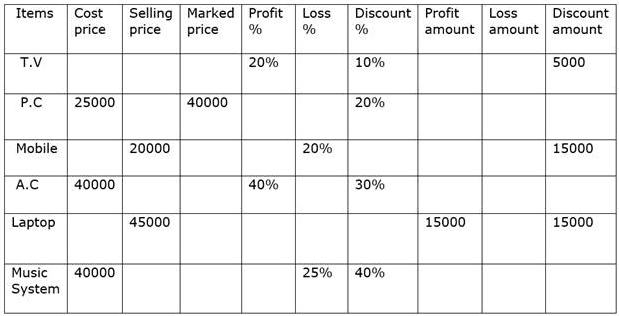Selling price of T.V is what percent of Marked price of laptop?

Solution:

Selling price of T.V = 5000 x 100 / 10 x 90 / 100 = 45000

Marked price of laptop = 45000 + 15000 = 60000

Required % = 45000/60000 x 100 = 75%

QUESTION: 9

Direction: In the given question below there are three statements followed by two conclusions numbered I and II. You have to take the given statements to be true even if they seem to be at variance with commonly known facts. Read all the conclusions and then decide which of the given conclusions logically follows from the given statements disregarding commonly known facts.

Statements:

All oils are sands

Some clays are oils

All clays are rocks

Conclusions:

I. At Least some clays are sands

II. Some oil is not rock

Solution:

Statements

All oils are sands

Some clays are oils

All clays are rocks

Combining all three statements, we get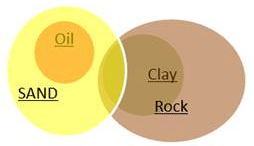Conclusions

I. At least some clays are sands

II. Some oil is not rock

Conclusion I

Some clays are oils + All oils are sands = Some clays are sands

Hence, Thus, the conclusion I follow.

Conclusion II

Some clays are oils → conversion → some oils are clays + All clays are rocks = Some oils are rock. Hence, conclusion II does not follow.

QUESTION: 10

Direction: In the given question, the 1st part of the sentence is given. The rest of the sentence/passage is split into four parts and named A, B, C and D. These four parts are not given in their proper order. Read the sentence and find out which of the four combinations is correct.

1) According to Indian Express, on Sunday, six men reached a South Delhi businessman's house in Malviya Nagar in a Tata Safari car bearing the Haryana government’s sticker fixed on the windscreen.

(A) One of the guys first took away all the cell phones of the businessman’s family members alleging that they had come on government duty to investigate a tax evasion charge.

(B) The plan could have been successfully executed but one of the family members found their behaviour suspicious.

(C) When the family member raised an alarm about the same, about 150 people from the same locality gathered outside the trader's house and the con men were beaten up and interrogated before they were handed over to the police.

(D) They “searched” the house, moving from room to room, and collected Rs 20 lakh in cash that they kept in their cars.

Solution:

The first sentence 1 introduced a situation, and the rest of the sentences should be arranged in the order that they happened. Clearly A is the first in sequence as it uses the word 'first' indicating the first step of these six men. The next step is described in D. Now, the twist comes in sentence C as the suspicions rise. People cannot get suspicious of something unless something actually happened. So, we place A first, followed by D and B. This leaves C for the last, which describes the result of those suspicions. Hence, the correct answer is d.

QUESTION: 11

Consider the following conditions:

1. tp < Δt

2. Δt < T

3. tp > Δt

4. Δt > T

Where, tp = pulse width,

Δt = propagation delay and

T = clock time period.

The race around condition in the Flip-Flop can be avoided if conditions

Solution: Condition to avoid Race around problem is tp < Δt < T.
QUESTION: 12

Consider the following schedules involving three transaction:

S1 : W2(x), W1(x), R3(x), W2(y), R3(y), R3(z), R2(x), R1(y)

S2 : R2(z), W2(x), W2(y), R1(x), R3(x), R2(z), R3(y), W1(x)

Which of the above schedules are conflict serializable?

Solution: A schedule is serializable if the precedence graph does not contain any cycle.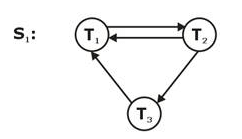It contains cycles so it is not serializable.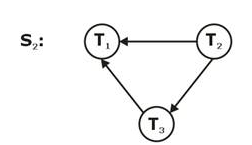It contains no cycle so is serializable.

So option (C) is correct.

QUESTION: 13

Consider the grammar defined by the following production rules:

S → A * C

A → B + A | B

B → A – B | A

A → id

B → id

C → id

Which of the following is true?

Solution: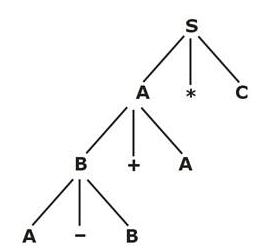+ and – are both right associative and + has higher precedence than*. So the correct option is (c).

QUESTION: 14

The output waveforms of a counter circuit shown below: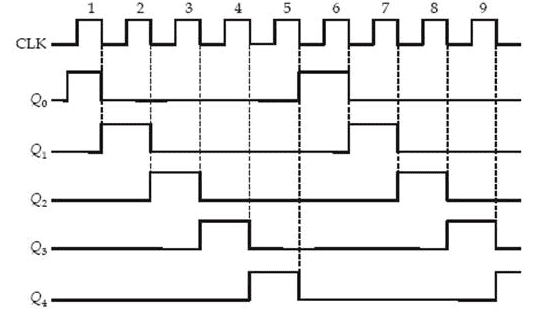The counter is

Solution: The waveform is of the ring counter because in one clock period only one flip-flop output is active and the same is repeating.
QUESTION: 15

For k number of users, how many keys are needed using private key cryptography and public key cryptography schemes respectively?

Solution:

In symmetric ⇒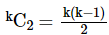Asymmetric ⇒ 2k

Explanation for asymmetric:

Every user has a (public key, private key) pair.

1 user ⇒ 2 keys

k user ⇒ 2k keys

Hence option (C) is the right answer.

QUESTION: 16

A binary operation Ηon a set of integers is defined as x⊙y=x2+y2+2xy. Which one of the following statements is true aboutΗ?

Solution: 1.Check for commutative

(x⊙y)=(y⊙x)

x2 + y2 + 2xy = y2 + x2 + 2yx

Since LHS = RHS

So ⊙ is commutative.

2. Check for associative:

(x⊙y)⊙z=x⊙(y⊙z)

(x2 + y2 + 2xy) Η z = x Η ( y2 + z2 + 2yz)

x4 + y. + 4x2y2 + 2x2y2 + 4xy3 + 4x3y + z2 + 2x2z + 2y2z + 4xyz ≠ x2 + y4 + z4 + 4y2z2 + 2y2z2 + 4y3z + 4yz3 + 2xy2 + 4xyz

So A is not associative.

QUESTION: 17

A token bucket scheme is used for traffic shaping. A new token is put into the bucket every 10 μsec. Assume each token picks one packet which contains 2 bytes of data. It is observed that the initial capacity of the bucket is 10 Mbits. The computer can transmit at the full speed of 8 Mbps for ________ (in seconds upto 2 decimal places)

Solution: We know,

C + R × S = M × S

Where, C = Initial capacity

R = Token rate

M = Output rate

S = Bursty traffic

Given, C = 10 Mbits

Token arrives at an interval of 10 μsec.

Then in 1 sec 100000 token arrives.

So, 100000 × 16 bit

= 16 × 105 bits/sec

= 1.6 Mbps

Hence R = 1.6 Mbps

Substituting we get

S =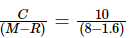= 1.5625

QUESTION: 18

The incorrect match (when n > 1) is

Solution: MD model has n-control units and since in multiprocessing, it requires n ALU units also.
QUESTION: 19

An Internet Service Provider (ISP) has a block 219.50.0.0/16. There are 3 groups, Group1 has 128 customers and each requires 64 IP addresses. Group2 has 64 customers and each requires 256 IP addresses. Group3 has X customers and each requires Y IP addresses. After successfully completing customer requests only 38K IP address left with (ISP)What can be the possible value of X and Y respectively.

Solution:

38 × 210 = [216 – [Group1 + Group2 + Group3]]

38 × 210 = [216 – [27 × 26 + 26 × 28 + 2m × 2n]]

2x × 2y = 216 – 213 – 214 – 38 × 210

= 210[26 – 23 – 24 – 38]

= 210[64 – 8 – 16 – 38] = 210 × 21

2x × 2y = 211

Option (d), which 26 × 25 = 211

QUESTION: 20

Let R be a relation on the set A = {1, 2, 3}, such that R = {(1,1), (2,2), (3,3), (1,2)}. Now consider the following relations.

I. {(1,1), (2,2), (3,3)}

II. {(1,1), (2,2)}

III. {(1,1), (2,2), (3,3), (1,2)}

Which of the above correctly represent(s) the smallest reflexive closure of R?

Solution: The common mistake here is to pick both I and III as the answer, which is wrong. Why? Because first of all in order for a relation T (say) to be reflexive closure of a relation R, then firstly T must be a superset of R, and only then we think about reflexivity, So I is actually a subset of R and thus cannot be the reflexive closure of R. II does not contain (3,3) and therefore is not reflexive, So the correct answer is choice (c).
QUESTION: 21

The sorting algorithm which requires least number of swaps in the worst case is

Solution:

Insertion Sort ⇒ O(n2)

Selection Sort ⇒ O(n)

Bubble Sort ⇒ O(n2)

Quick Sort ⇒ O(n2)

Amongst all these algorithms, selection sort requires the least number of swaps.

QUESTION: 22

We are given an array A in which every element is either 0 or 1. The time complexity of the most efficient algorithm which sorts A in descending order is equal to

Solution: The simplest way is to scan the entire array once, and maintain a count of the number of 0's (zero_count) and 1's in the array (one_count) - for every 0 encountered, increment the zero_count and similarly do the same for the 1's also.

And then overwrite the array by first filling the array with 1's - the number of 1's being equal to one_count value, and do the same for 0's also.

All this will take O(n) time and (A) is the answer.

QUESTION: 23

A 4-way set associative cache has lines of 32 byte and a total cache size of 16 KB. Which of the following main memory blocks is mapped onto the set ‘13’ of the cache memory when 16 MB of main memory is used?

Solution:

Number of lines =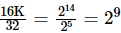Number of sets =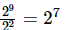Cache representation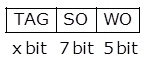Physical address size = 16 MB = 24 bit

24 = x + 7 + 5

x = 12 bit

Now, to map physical memory at 13th set bit (b11 b10 b9 b8 b7 b6 b,) MM address should be 13.

In option (d)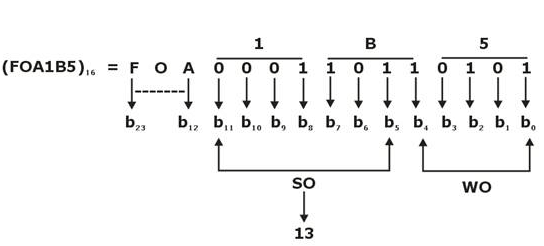Hence option (d) is correct.

QUESTION: 24

Consider the following relations, SQL query and given instances of relations: (where keys are underlined)

Student (snum, sname)

Enroll (snum, cname)

SELECT S.name FROM Student S WHERE

S.snum NOT IN (SELECT E.snum FROM Enroll E)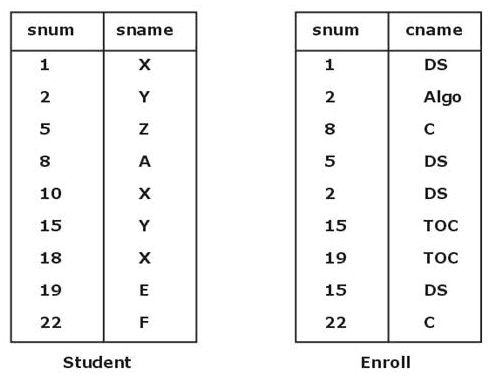Number of tuples returned by the SQL query is ________.

Solution:

SELECT S.Name FROM Student S WHERE

S.snum NOT IN (SELECT E.snum FROM Enroll E)

It return snum of all students who is enrolled in any course.

Query return the sname of a student who is not enrolled in any course.

SQL does not eliminates duplicate so relation given by SQL query is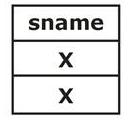Total 2 tuple returns.

QUESTION: 25

Consider two languages, L1and L2:

L1= {an| n > = 0} and L2 = {bn | n > = 0}

Which of the following correctly represents L1⋅ L2, where ‘⋅’ is the concatenation operation?

Solution:

L1 . L2 will be equal to {an bm| m , n > = 0}, which is same as {an bm| m = n, n > –1}.

QUESTION: 26

Number of binary trees formed with 5 nodes are

Solution: Possible number of binary trees formed when n number of nodes are given is 2nCn / n+1 so in this case the number of nodes are 5 so

Number of binary trees possible are = 10C5 / 6 = 10!/ 5! * 5! * 6 → 42

Hence option D is the correct answer

Total number of different Binary tree of size

5 : 14 + 5 + 4 + 5 + 14 = 42

QUESTION: 27

The Boolean function f implemented in the figure using two input multiplexers is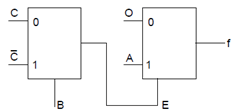Solution: f = E.A

E =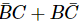∴ f =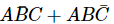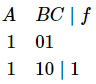QUESTION: 28

The number of colours required to properly colour the vertices of every planar graph is

Solution: According to the 4-colour theorem states that the vertices of every planar graph can be coloured with at most 4 colours so that no two adjacent vertices receive the same colour.

Hence, Option (C) 4 is the correct choice.

QUESTION: 29

Consider the following statements about simple connected undirected graph having more than 2 vertices:

1) At least two vertices have the same degree

2) At least three vertices have the same degree

Which one of the above statements hold true?

Solution: As the graph is simple, there is no self loop or parallel edge. As the graph is connected, no vertex can have 0 degree and hence, the degree range from 1 to n-1 which implies that the degree of at least two vertices must be the same.
QUESTION: 30

Given a combinational circuit below:

(2 X 1 multiplexer are being used)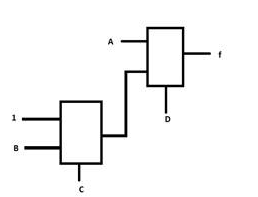What will be the sum minterms at output f?

Solution:

f = ( C’ + BC ) D + A D’

f = C’ D + BCD + AD’

Min-terms that represent f are

C’ D - 0 0 0 1, 0 1 0 1, 1 0 0 1, 1 1 0 1

B C D - 0 1 1 1, 1 1 1 1

A D’ - 1 0 0 0, 1 0 1 0, 1 1 0 0, 1 1 1 0

f = min-terms (1 , 5 , 7 , 8 , 9 , 10, 12 , 13 , 14 ,15)

Now

1 + 5 + 7 + 8 + 9 + 10 + 12 + 13 + 14 + 15 = 94.

QUESTION: 31

A bolt is manufactured by 3 machines A, B and C. Machine A turns out twice as many items as B, and machines B and C produce an equal number of items. 2% of bolts produced by A and B are defective and 4% of bolts produced by C are defective. All bolts are put into 1 stockpile and 1 is chosen from this pile. The probability that it is defective is ____.

Solution: Required probability - Chosen a defective bolt from Machine A + Chosen a defective bolt from Machine B + Chosen a defective bolt from Machine

=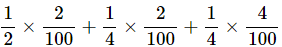= 0.025

QUESTION: 32

Which of the following operations is closed for the recursively enumerable languages?

Solution: Complement and Difference operations are not closed but Kleene closure is closed for RELs.
QUESTION: 33

From the following instance of relational schema R (A, B, C), We can conclude that: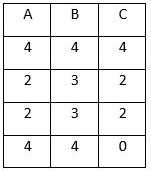Solution: A functionally determines B

B does not functionally determine, C because for B = 4, C has two values 4 and 0

A does not functionally determine, C because for A = 4, C has two values 4 and 0

QUESTION: 34

Consider the following three address code tables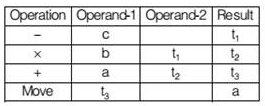Which of the following expression represents the above three address code (quadruple notation)

Solution:

t1=-c

t2=b ×t1

t3=a+t2

a=t3

⇒ a=a+b×(-c)

Option C is correct.

QUESTION: 35

Match the following groups.

List-I

A). Allocation

B). Relocation

List-II

I. Resolve the symbol references.

II. Alters the address of instructions and data.

III. Makes the program ready to execute by keeping the machine code in main memory.

IV. Assigns the required memory space for the program.

Solution:

Allocation: Loader allocates the required memory space for the program to execute properly.

Relocation: It can alter the address of instructions and data during execution.

QUESTION: 36

Given a relation is in 3NF, Which of the following can be inferred from this?

Solution:

Each non key attribute is determined by the super key,

A relation C-> D is in 3NF if,

1) C is a super key

or

2) D is a prime attribute

If D is a non prime attribute then C must be a super key.

QUESTION: 37

For the given instructions, all type of data dependencies(RAW, WAW, WAR) are present.

STORE R1, 0[R2]

STORE R3, -8[R9]

Then which of the following is true?

Solution:

From the given instructions clearly,

- RAW exists if (R2+0) == (R8+24)

– WAR exists if (R8+24) == (R9 – 8)

– WAW exists if (R2+0) == (R9 – 8)

QUESTION: 38

An AVL tree is constructed by inserting the following sequence of elements into empty AVL tree. After building an AVL tree, if an element ‘4’ is deleted from it, find how many levels are present in the AVL tree.

7, 4, 9, 5, 4.5, 6, 5.5, 5.2

Solution:

7, 4, 9, 5, 4.5, 6, 5.5, 5.2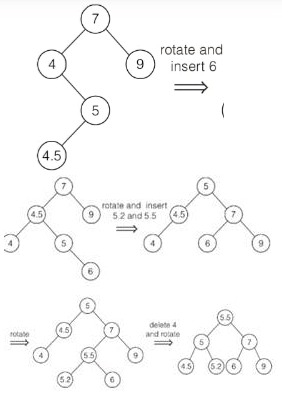Thus, 3 levels are present in the final AVL tree.

QUESTION: 39

A state diagram of a logic which exhibits a delay in the output is shown in the figure, where X is the do not care condition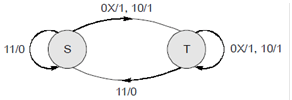The logic gate represented by the state diagram is

Solution: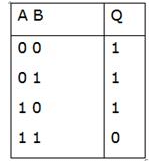If any one of the inputs is zero, output is logic ‘1’. Otherwise output is logic ‘0’, which represents the NAND gate.

QUESTION: 40

There are five buildings A, B, C, D, E in a row. You are given the following statements:

E is to the east of C and west of A A is to the west of B

B is to the west of D

Which building is in the middle?

Solution:

As E is east of C and westof A: Hence, C E A A is to the west of B: C E A B

B is the west of D: C E A B D

QUESTION: 41

Four channels are multiplexed using TDM. If each channel sends 100 bytes/second and we multiplex 1 byte per channel, then the bit rate for the link is ……………bps

Solution:

Firstly we understand the working of Multiplexer using TDM.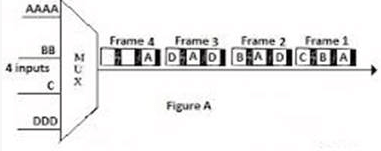Each frame contain 1 byte from each channel; { 1 Byte = 8 Bit}

we have 4 channels

Then ,the size of each frame is

1x4= 4 bytes = =~ 32 bits.

Each channel is sending 100 bytes/second

A frame carries 1 byte from each channel,

The frame rate must be 100 frames per second. { its given}

Then the total rate is = 32×100 = 3200 bits/second

QUESTION: 42

If it takes 50ns to search associative registers and also about 100ns to get access to main memory then find out the % of slowdown in memory access time? (Given hit ratio 90%).

Solution:

Effective access time = (hit ratio) + (memory access time + search time in associative registers) + (fail ratio) × (search time in associative registers)

= (0.90 × 150) + (0.10 × 250)

=160 ns

% slowdown = 160-100= 60 ns.

QUESTION: 43

#include

void main(){

int k,s=0;

int arr[]={5,9,2,6,0,1,8};

for(int k=0;k<7;k++)

{

if(((int)str[k]%2)==0)

printf("%c%d",(int)str[k],arr[k]);

}

}

What will be the output of the above code?

Solution:

if(((int)str[k]%2)==0) // if ASCII value of character of char array str is even then if condition is true

(int)str[k]=> indicates ASCII value of character of character array str

In string “Gradeup”, only r, d, p characters have even ASCII value,so these are printed, along with this element of int array arr having same index to that of character having even ASCII value is also printed.

QUESTION: 44

Consider the C program below.

#include int *A, stkTop;

int stkFunc(int opcode, int val)

{

static int size=0, stkTop=0;

switch (opcode) {

case -1: size = val; break;

case 0: if (stkTop < size) A[stkTop++] = val; break; default: if (stkTop) return A[--stkTop];

}

return -1;

}

int main()

{

int B; A = B; stkTop = -1;

stkFunc (-1, 10);

stkFunc ( 0, 5);

stkFunc ( 0, 10);

printf ("%d\n", stkFunc(1, 0) + stkFunc(1,0));

}

The value printed by the above program is ___________.

Solution:

The code in main, basically initializes a stack of size 10, then pushes 5, then pushes 10. Finally the printf statement prints the sum of two pop operations which is 10 + 5 = 15.

stkFunc (-1, 10); // Initialize size as 10

stkFunc (0, 5); // push 5

stkFunc (0, 10); // push 10

// print sum of two pop

printf ("%d\n", stkFunc(1, 0) + stkFunc(1, 0));

QUESTION: 45

Consider the following set of relation schemas

STUDENTS (ROLLNO, NAME, DOB, AGE)

ENROLL (ROLLNO, COURSENO)

COURSES (COURSENO, COURSENAME, INSTRUCTOR)

Consider the following FD’s and tell the normal form in which the relations exist.

FD1: DOB →AGE

FD2: NAME →ROLLNO

FD3: ROLLNO →NAME

FD4: COURSENO →COURSENAME

FD5: COURSENO →INSTRUCTOR

FD7: ROLLNO →COURSENO

FD8: COURSENO →ROLLNO

Solution:

(ROLLNO, DOB), (NAME, DOB) and (ROLLNO, COURSENO) are the candidate keys.

The DOB →AGE is a partial dependent FD for the candidate key. So, the relation is in 1NF.

Thus, none of the given options are true.

QUESTION: 46

AB + tree index is to be built on the name attribute of the relation ‘student’. Assume that all the students' names are of length 8 bytes, disk blocks are of size 512 bytes and index pointers are of size 14 bytes. Given this scenario that would be the best choice of the degree (no. Of pointers per node) of the b+ tree?

Solution:

order of the internal node is P1.

CP + (P1 - 1) ×KV <= BLOCK SIZE

Child Pointer (CP) = 8 bytes (students name is a child pointer)

Key Value (KV) = 14 bytes

Block Size = 512 bytes

8 + (P1 - 1) × 14 = 512

P1 = 37

QUESTION: 47

Consider the relation

Students(Rollno, Name, Marks)

Where rollno is the primary key.

Now we would like to rank the students according to the decreasing marks. The student with the highest marks gets rank 1. Ties are not broke but ranks are skipped.

Now consider 2 queries.

Query1: SELECT A .Name, count (B .Name)

From Students A, Students B

Where A.Marks <= B.Marks group by A .Name

Query2: SELECT A .Name, 1+count (B .Name)

From Students A, Students B

Where A.Marks < B .Marks group by A .Name

Solution:

Both the queries generate the same result but none of them sort the students according to the decreasing marks.

QUESTION: 48

Consider the following set of relation schemas

STUDENTS (ROLLNO, NAME, DOB, AGE)

ENROLL (ROLLNO, COURSENO)

COURSES (COURSENO, COURSENAME, INSTRUCTOR)

Consider the following SQL Query: -

Select distinct Name, Rollno

Which of the following sets is computed by the above query?

Solution:

Use of distinct selects Name and rollno only once. So, irrespective of how many times a person has got an A grade, his name would appear only once in the output.

QUESTION: 49

Regular languages are

Solution:

According to Chomsky hierarchy, regular languages come under type 3 i.e. level 3 language class.

QUESTION: 50

Which of the following is correct output for the program code given below?

main( )

{

void pr( );

pr ( );

pr ( );

pr ( );

void pr ( )

{

static int i = 1;

printf (“%c”, (65+ i ++));

}

}

Solution:

The correct output is “BCD” when the function pr ( ) is first called the value of i is initialized to 1.

After the pr ( ) completes its execution i = 2 is retained for it’s next call as “i” is a static variable.

∴ 65 + 1 = 66 = B

65 + 2 = 67 = C

65 + 3 = 68 = D

∴ BCD is the correct output.

QUESTION: 51

Which switching technique is used in the telephone network?

Solution:

Circuit switching technique is used in telephone networks. In this technique a dedicated network path is established for communication, for this, when two persons talking on telephone through one network path, no one else can use that path even if no talking (means no signal transfer through that path) is done.

QUESTION: 52

A hash table of length 10 is taken for insertion of the keys with hash function h(key)= key mod 10. If Collision occurs, it is resolved with quadratic probing. The following sequence of keys are inserted:

46, 36, 34, 24, 52, 57, 56

Which of the following cannot be inserted to the table due to quadratic probing?

Solution:

Insertion of 46, H(46)= 46 mod 10= 6, inserted at 6th position

Insertion of 36, H(46)= 46 mod 10= 6,

Collision occurs, hence, quadratic probing is applied,

QP (key, I)= (h(key)+i+ i2) mod m

At i=1, QP (36, 1) = 8

So 36 will be inserted at 8.

Insertion of 34: It can be inserted at place 4 in the hash table.

Insertion of 24:h(24)= 24 mod 10= 4

Collision will occur for key 24. For resolving the collision, Quadratic probing is resolved

QP (24, 1)= 6, again Collision

QP (24, 2)= 0 o collision is resolved.

QUESTION: 53

Match the following: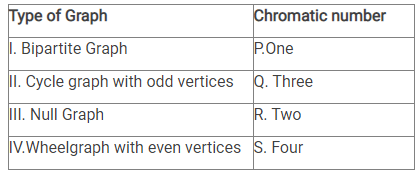Which option is correct :

Solution: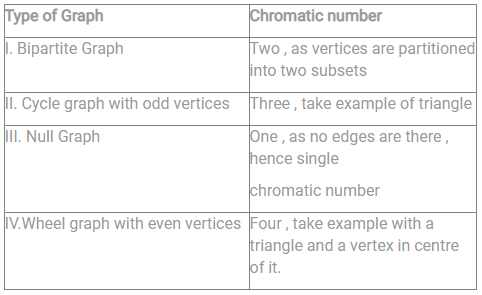QUESTION: 54

A wireless network interface controller can work in-

Solution:

An 802.11 WNIC can operate in two modes known as ad hoc mode and infrastructure mode. In an ad hoc mode network the WNIC does not require an access point, but rather can interface with all other wireless nodes directly. All the nodes in an ad hoc network must have the same channel and SSID. Infrastructure mode is an 802.11 networking framework in which devices communicate with each other by first going through an Access Point (AP). In infrastructure mode, wireless devices can communicate with each other or can communicate with a wired network.

Hence, the correct option is (c).

QUESTION: 55

Find the value of common digit (in decimal system) in 15's and 16's complement of (2E0)H

Solution:

First find 15's complement, for that subtract all digits by F

FFF

-2E0

---------

D1F

---------

Now to find 16's complement, add 1

D1F

+ 1

---------

D20

----------

So in both above results, 'D' is common whose value in decimal system is 13

QUESTION: 56

A B-Tree used as an index for a large database table has six levels including the root node. If a new key is inserted in this index, then the minimum number of nodes that could be newly created in the process are

Solution:

No nodes will be newly created if the new node is simply placed on the leaf level. Since the minimum is asked, it will be 0.

QUESTION: 57

The memory access time is 1 nanosecond for a read operation with a hit in cache, 5 nanoseconds for a read operation with a miss in cache, 2 nanoseconds for a write operation with a hit in cache and 10 nanoseconds for a write operation with a miss in cache. Execution of a sequence of instructions involves 100 instruction fetch operations, 60 memory operand read operations and 40 memory operand write operations. The cache hit-ratio is 0.The average memory access time (in nanoseconds) in executing the sequence of instructions is __________.

Solution:

Total instruction = 100 instruction fetch operation + 60 memory operand read operation + 40 memory operand write op

= 200 instructions (operation)

Time taken for fetching 100 instructions (equivalent to read)

= 90 × 1ns + 10 × 5 ns = 140 ns

Memory operand Read operations = 90% (60) × lns + 10% (60) × 5ns

= 54ns + 30ns = 84 ms

Memory operands write operation time = 90%(40) × 2ns + 10%(40) × 10ns

= 72ns + 40ns = 112 ns

Total time taken for executing 200 instructions = 140 + 84 + 112 = 336 ns

∴ Average memory access time = 336 ns/200 = 1.68ns

QUESTION: 58

The order of an internal node in a B+ tree index is the maximum number of children it can have. Suppose that a child pointer can take 6 Bytes, the search field value takes 14 Bytes and the block size is 512 Bytes. What is the order of the internal node?

Solution:

The order of internal node is = 6*n + (n-1)*14 ≤ 512 = 6n + 14n – 14 ≤ 512

= 20n ≤ 528 = n ≤ 26

QUESTION: 59

Let us say you positive integers a and b are taken from the user and then they are fed to the following function:

void function(int a, int b){

while( a != b){

if( a > b )

a = a - b;

else

b = b - a;

}

printf(“%d”, b);

}

What does the above function compute?

Solution:

This method is Euclid’s algorithm of finding GCD of two numbers. It is based on the fact that the GCD of two numbers doesn’t change if you replace the larger number with its difference with the smaller number.

QUESTION: 60

Consider the values of A = 2.0 × 1030, B = -2.0 × 1030, C = 1.0, and the sequence

X: = A + B Y:= A + c

X: = X + C Y:= Y + B

Executed on a computer where floating point numbers are represented with 32 bits. The values for X and Y will be

Solution:

The given input is a 32-bit representation. The maximum precision value will be 32 bits. It has 10 digits.

A = 2.0 × 1030

B = -2.0 × 1030

C = 1.0,

If we add the value A and C means we will get 31st digit to 1, which will go outside the level of A. This addition’s result will return the A value which is assigned to Y. Y+B result is 0.0. X+C result will return 1.0. So option B is the Solution.

QUESTION: 61

Identify the minimal product-of-sums function described by the K-map given in the figure. Where ϕ is don’t care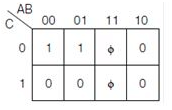Solution: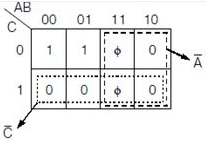A’C’ is the minimal POS

QUESTION: 62

Let A= 1100 1010 and B= 0000 0100 be two 8-bit 2's complement numbers. Their product in 2's complement is

Solution:

A= 1100 1010, since MSB=1 hence the number is negative.

2’s complement of A= 00110110

A= -54

B= 0000 0100

B= 4 (Since the number is positive so there is no 2’s complement).

Now product of A*B= -54*4 = 216.

Binary representation of -216= 1101 1000.

2’scomplement of -216= 0010 1000.

QUESTION: 63

How many of the following statements are true?

1) Function must be neutral function is a necessary as well as sufficient condition for a function to be a dual function

2) For a n variable function, there are 2n-1 mutually exclusive pairs.

3) f(x,y,z) = Σm( 1 , 2 , 4 , 6 ) is a dual function.

4) Dual functions are a subset of Neutral functions.

Solution:

Only statements b, d and e are correct statements. As a function should be a neutral function, to be a dual function is only a necessary condition, not the sufficient one.

QUESTION: 64

Consider the following data: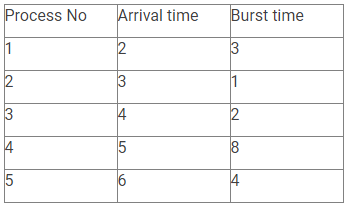Suppose round-robin scheduling is applied to the given data with time quantum=2 units. It is also given that scheduling of a process or context switch will take 1 unit of time. What are the completion time and turnaround time of process P4?

Solution: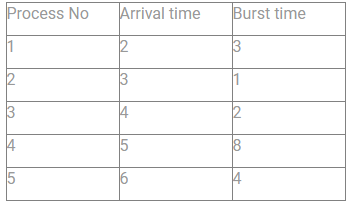Here, TQ = 2 units

Also, every scheduling OT context switch will take the overhead of 1ns. (Assume & represent overhead)

The Gant Chart will look like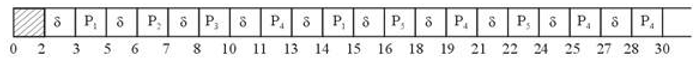Hence, completion time = 30 units

& twin around time = completion time – Arrival time

= 30 – 5

= 25 units

QUESTION: 65

Which of the following flip-flops is used to avoid race around problems?

Solution:

Master-Slave flip flop is free used to avoid the race around condition.Use Code STAYHOME200 and get INR 200 additional OFF Use Coupon Code